# 感知机：教程，实现和可视示例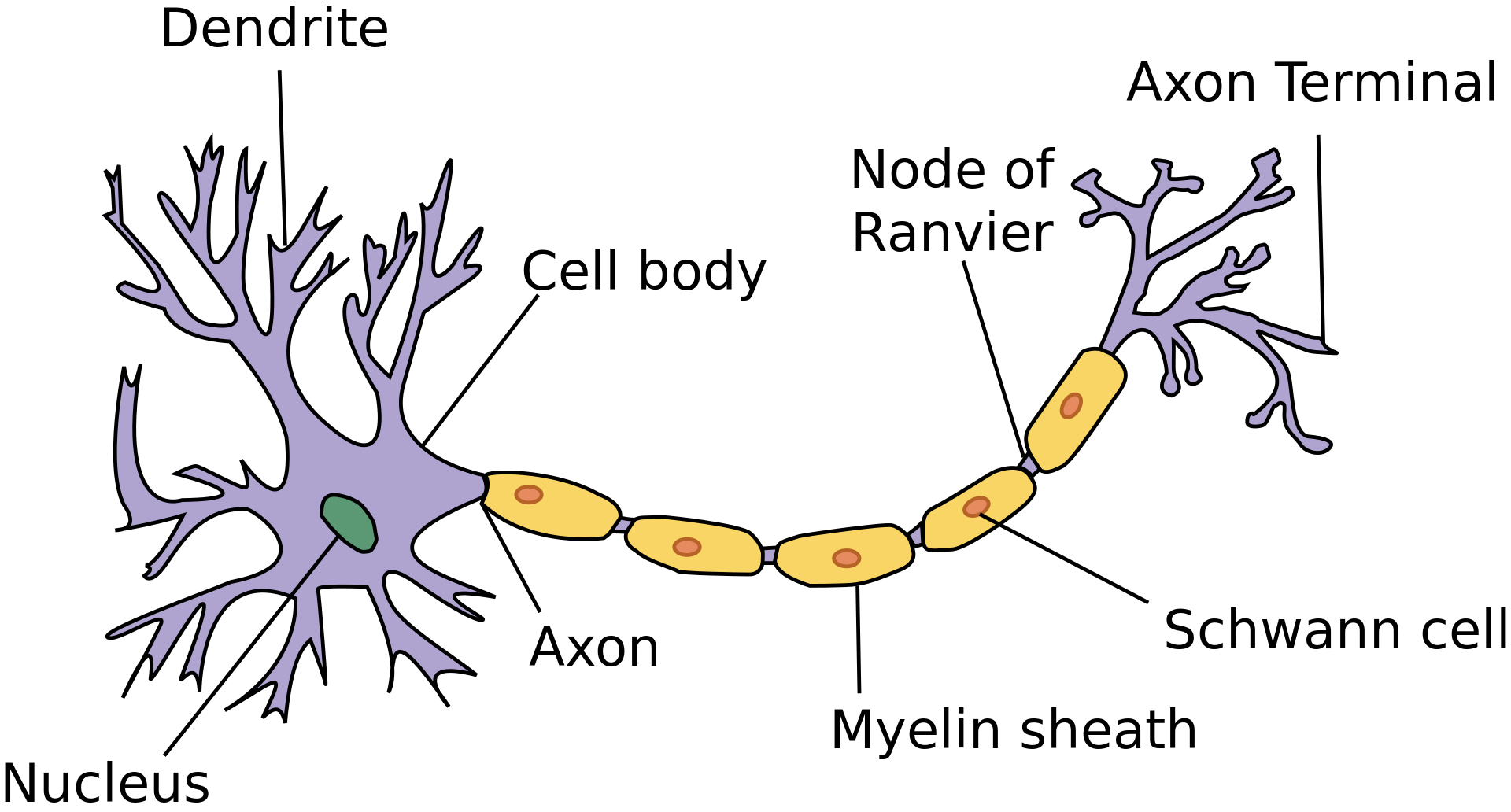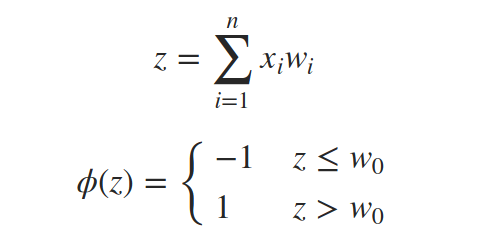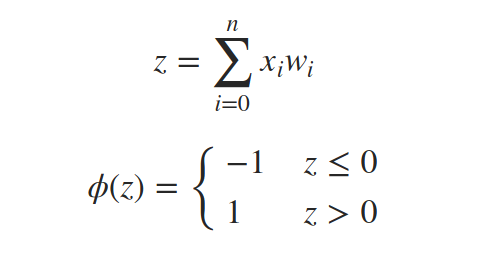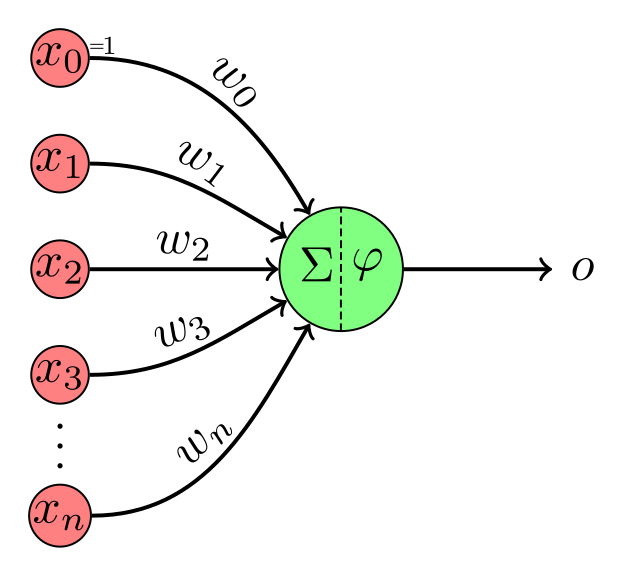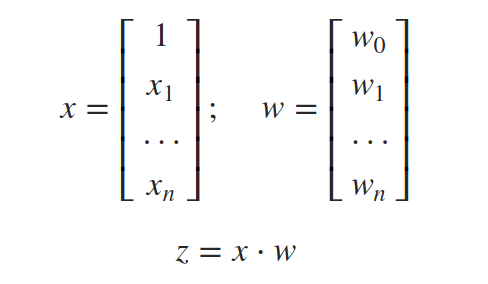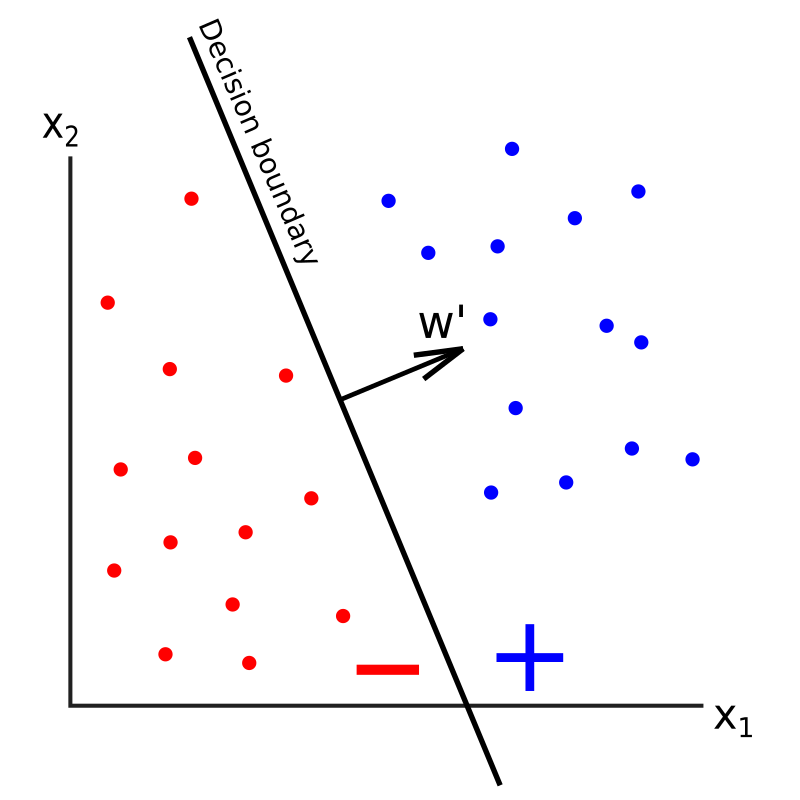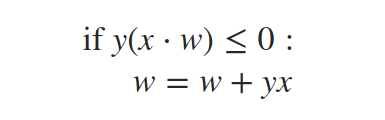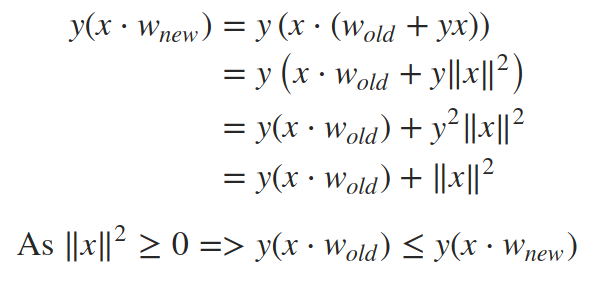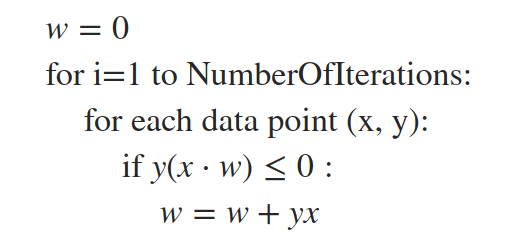### Python实现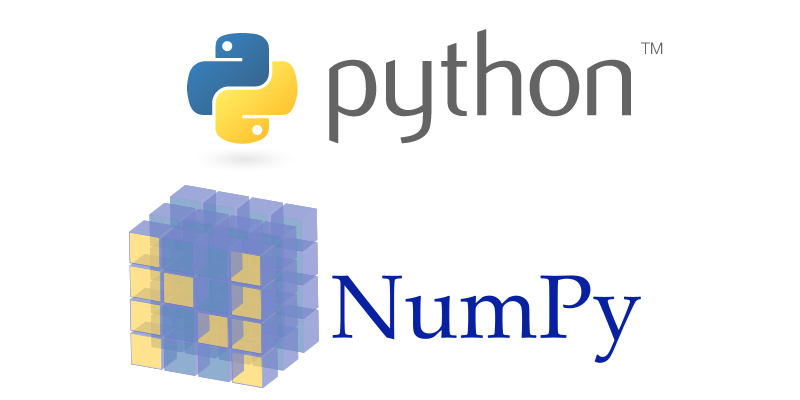.fit()方法将用于训练感知器。它期望第一个参数是2D numpy数组X。该数组的行是数据集的样本，列是特征。第二个参数y应该是1D的numpy数组，它包含X中每行数据的标签。第三个参数n_iter是我们让算法运行的迭代次数。

def fit(self, X, y, n_iter=100):

n_samples = X.shape
n_features = X.shape

# 偏置都加1
self.weights = np.zeros((n_features+1,))

X = np.concatenate([X, np.ones((n_samples, 1))], axis=1)

for i in range(n_iter):
for j in range(n_samples):
if y[j]*np.dot(self.weights, X[j, :]) <= 0:
self.weights += y[j]*X[j, :]


.predict()方法将用于预测新数据的标签。它首先检查weights对象属性是否存在，如果不存在，则表示感知器尚未训练，然后显示警告消息并返回。该方法需要一个与.fit()方法形状相同的参数X。然后我们在X和权重之间做一个矩阵乘法，然后把它们映射到-1或+1。我们使用np.vectorize()将此映射应用于矩阵乘法结果向量中的所有元素。

def predict(self, X):
if not hasattr(self, 'weights'):
print('The model is not trained yet!')
return

n_samples = X.shape
X = np.concatenate([X, np.ones((n_samples, 1))], axis=1)
y = np.matmul(X, self.weights)
y = np.vectorize(lambda val: 1 if val > 0 else -1)(y)

return y


score()方法计算并返回预测的准确性。它期望输入矩阵X和标签向量y作为参数。

def score(self, X, y):
pred_y = self.predict(X)

return np.mean(y == pred_y)


### 几个例子

X, y = make_classification(
n_features=2,
n_classes=2,
n_samples=200,
n_redundant=0,
n_clusters_per_class=1
)


X, y = make_circles(n_samples=200, noise=0.03, factor=0.7)


#### 例1 线性可分的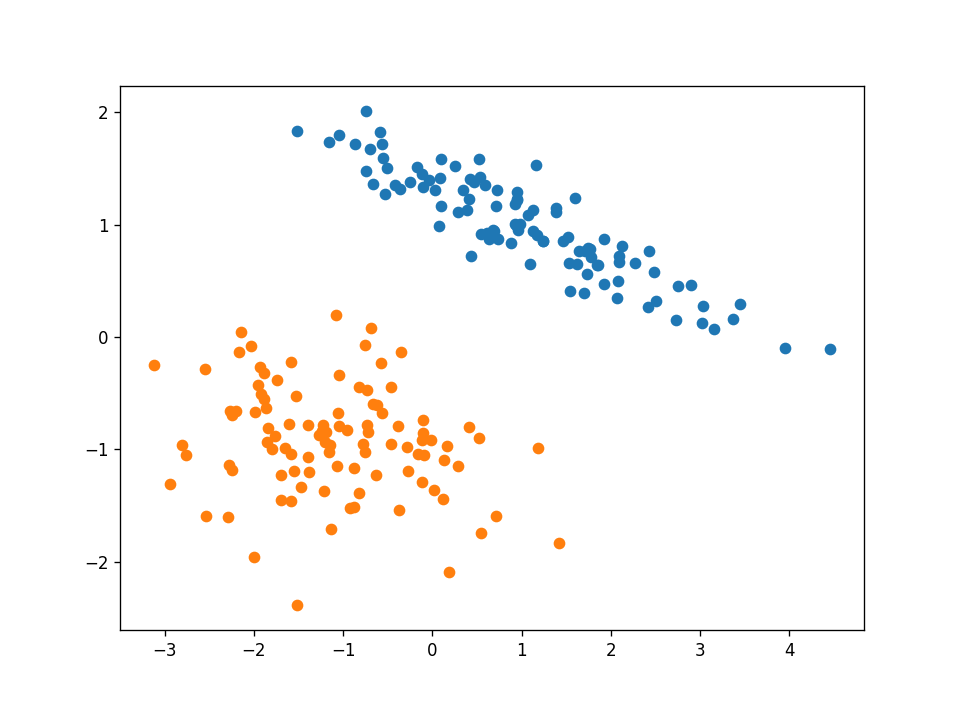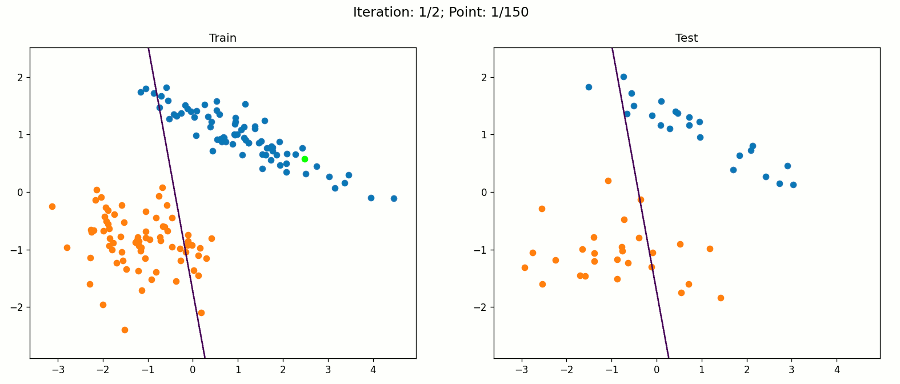#### 例2 噪声数据集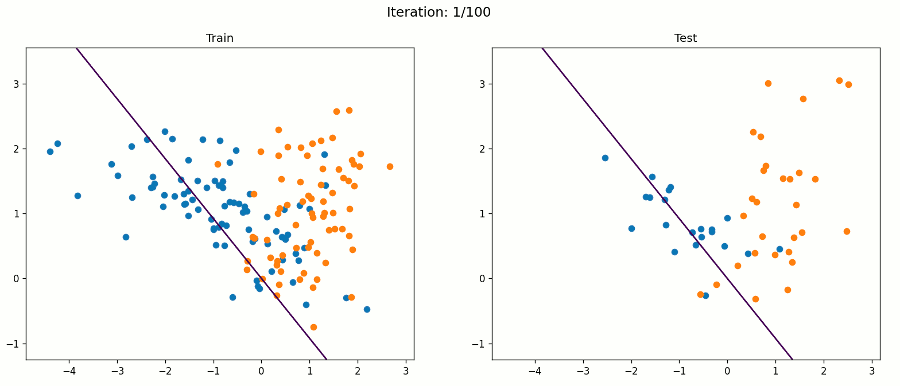#### 例3 非线性数据集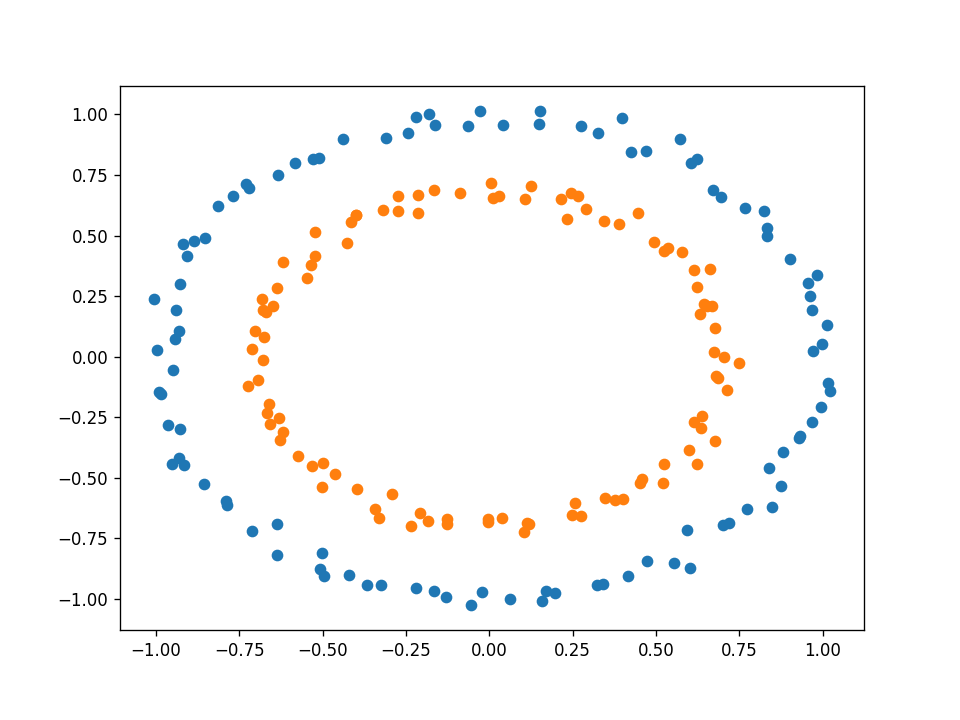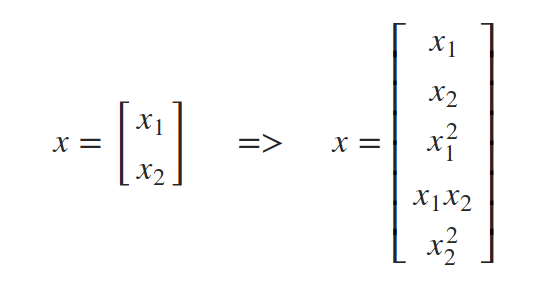def polynom(indices_list, indices, a, b, p):
indices = [*indices]
if p == 0:
indices_list.append(indices)
return
for i in range(a, b):
indices.append(i)
polynom(indices_list, indices, i, b, p-1)
indices = indices[0:-1]

def polynomial_features(X, p):
n, d = X.shape
features = []
for i in range(1, p+1):
l = []
polynom(l, [], 0, d, i)
for indices in l:
x = np.ones((n,))
for idx in indices:
x = x * X[:, idx]
features.append(x)
return np.stack(features, axis=1)


X = polynomial_features(X, 2)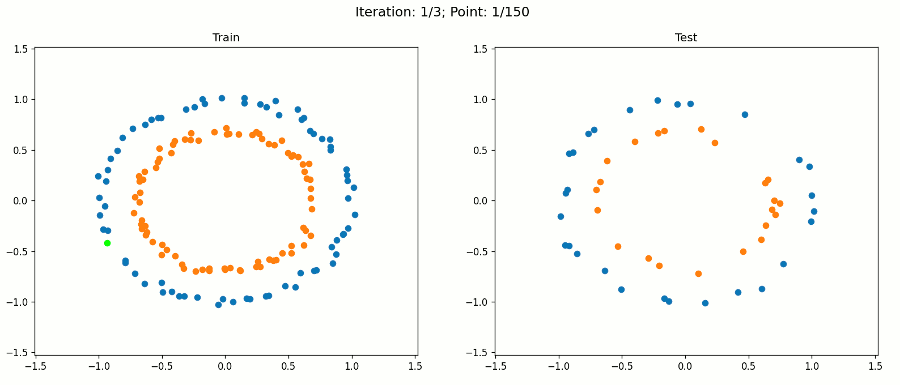http://panchuang.net/

sklearn机器学习中文官方文档：
http://sklearn123.com/

http://docs.panchuang.net/

posted @ 2020-07-08 01:27  人工智能遇见磐创  阅读(195)  评论(0编辑  收藏